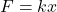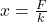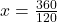## Two spherical point charges each carrying a charge of 40 μC are attached to the two ends of a spring of length 20 cm. If its spring constant

Question

Two spherical point charges each carrying a charge of 40 μC are attached to the two ends of a spring of length 20 cm. If its spring constant is 120 Nm-1 , what is the length of the spring when the charges are in equilibrium?

in progress 0
7 months 2021-07-12T11:26:40+00:00 1 Answers 0 views 0

## Answers ( )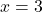Explanation:

From the question we are told that:

Charge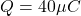Length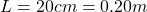Spring constant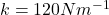Generally the equation for Force between Charges is mathematically given by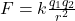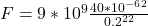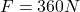Therefore